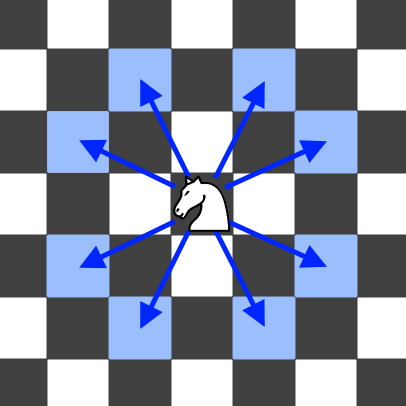0688. 骑士在棋盘上的概率

# 0688. 骑士在棋盘上的概率#

• 标签：动态规划
• 难度：中等

## 题目大意 #• $1 \le n \le 25$。
• $0 \le k \le 100$。
• $0 \le row, column \le n$。

 1 2 3  输入：n = 3, k = 2, row = 0, column = 0 输出：0.0625 解释：有两步(到(1,2)，(2,1))可以让骑士留在棋盘上。在每一个位置上，也有两种移动可以让骑士留在棋盘上。骑士留在棋盘上的总概率是 0.0625。 

## 解题思路 #

### 思路 1：动态规划 #

###### 3. 状态转移方程 #

• 假设下一步的落点为 (new_i, new_j)。从当前步选择 8 个方向其中之一作为下一步方向的概率为 $\frac{1}{8}$。
• 而每个方向上落点仍在棋盘内的概率为 dp[new_i][new_j][p - 1]。所以从 (i, j) 走到 (new_i, new_j) 的可能性为 $dp[new_i][new_j] \times \frac{1}{8}$。

###### 4. 初始条件 #
• 从位置 (i, j) 出发，移动不超过 0 步的情况下，最后仍留在棋盘内的概率为 1

### 思路 1：动态规划代码 #

  1 2 3 4 5 6 7 8 9 10 11 12 13 14 15 16 17 18 19  class Solution: def knightProbability(self, n: int, k: int, row: int, column: int) -> float: dp = [[[0 for _ in range(k + 1)] for _ in range(n)] for _ in range(n)] for i in range(n): for j in range(n): dp[i][j] = 1 directions = {(-1, -2), (-1, 2), (1, -2), (1, 2), (-2, -1), (-2, 1), (2, -1), (2, 1)} for p in range(1, k + 1): for i in range(n): for j in range(n): for direction in directions: new_i = i + direction new_j = j + direction if 0 <= new_i < n and 0 <= new_j < n: dp[i][j][p] += dp[new_i][new_j][p - 1] / 8 return dp[row][column][k] 

### 思路 1：复杂度分析 #

• 时间复杂度：$O(n^2 * k)$。外三层循环的时间复杂度为 $O(n^2 * k)$，内层关于 directions 的循环每次执行 8 次，可以看做是常数级时间复杂度。
• 空间复杂度：$O(n^2 * k)$。用到了三维数组保存状态。